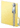Psychometric Toolbox
Reliability Analisys

Description

Function Classical Item Analysis --> CTT-based item analysis and reliability estimation.

The CIA function (a) performs classical test theory (CTT) item and scale analysis, and (b) estimates the reliability of the raw scores on the basis of Cronbach’s alpha coefficient. The CIA function contains two computing buttons. The "Compute" button performs CIA for a set of items intended to measure a single construct (i.e. single scale analysis). The "Compute Sub-Tests" button performs CIA for all the Sub-Tests created with the Data Preprocessing function.

In both cases the output is organized in two parts. The first part provides the descriptive analysis of the item and test scores. At the item level, the reported statistics are: (a) the inter-item correlation matrix, (b) the item means and standard deviations, and (c) the descriptive statistics for the test scores.

The second part of the output provides the item discrimination indices and the estimated reliability of the raw scores. The item indices are: (a) the corrected item-total correlation, (b) the squared multiple correlation, and (c) the alpha estimate if the item is deleted from the scale. The reliability estimates are: (a) the coefficient alpha based on the raw scores, (b) the corresponding 90% confidence interval, and (c) the standardized alpha.

Download example data file:Classical_item_analysis.zip